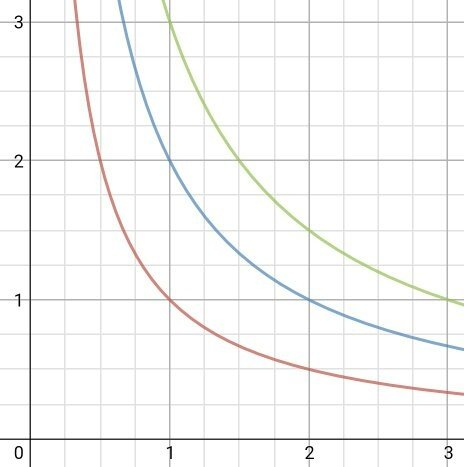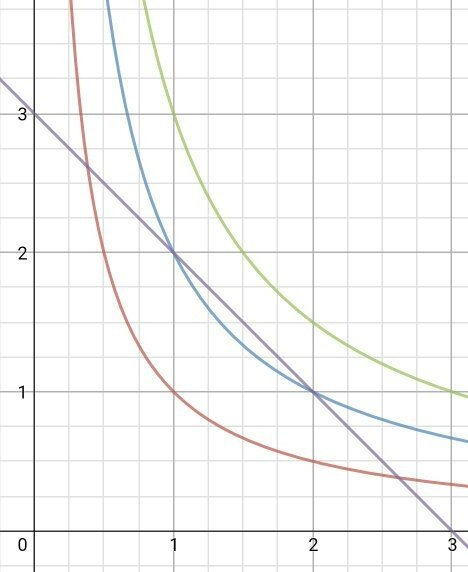# How to Solve Questions Quickly in IIT JEE and NEET?

By | Last updated on September 16th, 2020Speed is an important factor in clearing exams like IIT JEE and NEET. Keeping an eye on accuracy, aspirants need to learn how to solve questions quickly in JEE and NEET. This comes with a lot of practice and study.

## How to Solve Questions Quickly in IIT JEE and NEET?

Here are the tips to solve questions quickly in JEE and NEET:

1. Remember all the formulas of Physics, Chemistry and Math well. If you take time to recall the formula, you will lose the time.
2. Try to get hints from questions and options, which chapter or topic or concept is asked. Because JEE and NEET will have questions from almost all chapters of the syllabus.
3. Try to solve options. (Be careful, it’s a risky way. You may end up answering an easy question in a wrong way.) But, it may be useful at times.
4. Think for another method to solve the question. It may be a shortcut which might be valid only for that particular question (due to some special symmetry). Such tricks will solve your question in 1 minute instead of 4–5 minutes.

One such question (related to point 4) was asked in JEE main 2016. It had a straight line graph between Pressure and volume of a gas. We had to find the maximum temperature of an ideal gas during undergoing this process. The usual method is to find T as f(V) or f(P), then equating derivative of T =0.

Another approach (that I found out during the exam) is to think about PV=constant graphs at different temperatures, which will help you realise that the hyperbola shifts “upward” along line P=V as temperature increases.So, the given PV line will touch the “highest” possible hyperbola at the midpoint of its intersections with x and y axes. Hence, the maximum temperature will occur at the midpoint for the given line. (By chance that line had slope = -1.)Learn some exclusive tricks to solve questions quickly from the best experts in JEE/ NEET Online Courses.

Related Posts :

How to Improve Speed and Accuracy for JEE/NEET?

What If Solving Questions Takes Too Much Time?We’re listening to students.